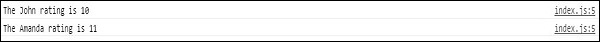# Firebase - Queries

Firebase offers various ways of ordering data. In this chapter, we will show simple query examples. We will use the same data from our previous chapters.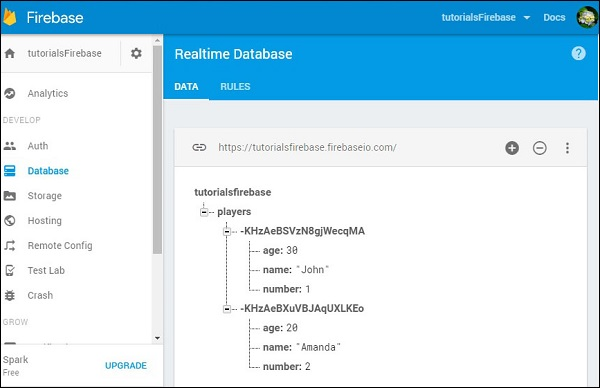## Order by Child

To order data by name, we can use the following code.

### Example

Let us consider the following example.

```var playersRef = firebase.database().ref("players/");

console.log(data.val().name);
});
```

We will see names in the alphabetic order.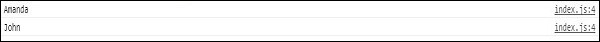## Order by Key

We can order data by key in a similar fashion.

### Example

Let us consider the following example.

```var playersRef = firebase.database().ref("players/");

console.log(data.key);
});
```

The output will be as shown below.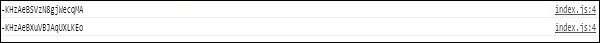## Order by Value

We can also order data by value. Let us add the ratings collection in Firebase.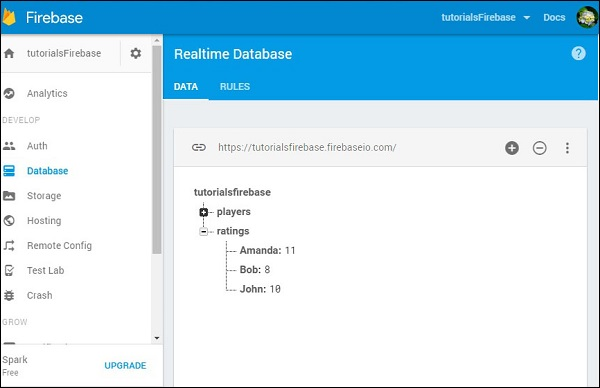Now we can order data by value for each player.

### Example

Let us consider the following example.

```var ratingRef = firebase.database().ref("ratings/");

ratingRef.orderByValue().on("value", function(data) {

data.forEach(function(data) {
console.log("The " + data.key + " rating is " + data.val());
});

});
```

The output will be as shown below.